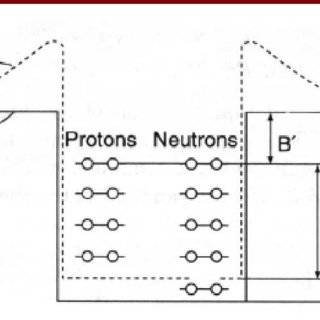# Fermi Gas Model

• I
lukka98In the fermi gas model, there is assumption that there is a 3D potential well, but there is "energetic degeneration" for each three index "nx, ny, nz".
Now the problem is with that image, if there is degeration, for some level En there may be 10 distinctive state with same energy, so there is 20 proton and 20 neutron for Pauli exclusion in that state, why in the image there are only two particle for each state?

Is because is a 1D potential well, just for simplify?

thanks